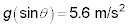##### Physics I Workbook For Dummies with Online Practice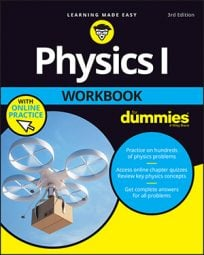When you have a block of ice (read: frictionless) moving down a ramp, it’s being acted on by forces, which means that it’s accelerated. How fast is it being accelerated? When you know that F = ma, you can solve for the acceleration.

After you solve for the force along the ramp, you can get the acceleration (a = F/m) along the ramp. Your block of ice is going to accelerate down the ramp.

## Sample question

1. Suppose you have a block of ice on a ramp at 40 degrees, and it slides down. What is its acceleration?

The correct answer is 6.3 m/s2.

1. What’s important here is the force along the ramp: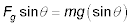2. The acceleration of the ice is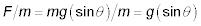In other words, the acceleration is the component of g acting along the ramp. Note that this result is independent of mass.

3. Plug in the numbers: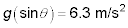Notice that you did not need to know the mass of the ice in this problem because the mass cancels out of the equation.

## Practice questions

1. Suppose that a block of ice is on a ramp with an angle of 60 degrees. What is its acceleration?

2. You’re unloading a couch on a cart from a moving van. The couch gets away from you on the 27 degrees ramp. Neglecting friction, what is its acceleration?

3. 3.You have a block of ice with a mass of 10.0 kg on a ramp with an angle of 23 degrees when it slips away from you. What is its acceleration down the ramp?

4. You’re sliding down a toboggan run at 35 degrees. What is your acceleration?

Following are answers to the practice questions:

1. 8.5 m/s2

1. The force along the ramp is2. The acceleration of the ice is3. Plugging in the numbers gives you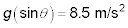2. 4.4 m/s2

1. The force along the ramp is2. The acceleration of the cart is3. Plugging in the numbers gives you g(sin theta) = 4.4 m/s2.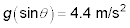3. 3.8 m/s2

1. The force along the ramp is2. The acceleration of the ice is3. Plugging in the numbers gives you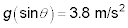4. 5.6 m/s2

1. The force along the ramp is2. Your acceleration is3. Plugging in the numbers gives you